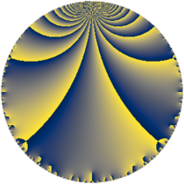# Properties

 Label 98.4.cLevel $98$ Weight $4$ Character orbit 98.c Rep. character $\chi_{98}(67,\cdot)$ Character field $\Q(\zeta_{3})$ Dimension $20$ Newform subspaces $8$ Sturm bound $56$ Trace bound $3$

# Related objects

## Defining parameters

 Level: $$N$$ $$=$$ $$98 = 2 \cdot 7^{2}$$ Weight: $$k$$ $$=$$ $$4$$ Character orbit: $$[\chi]$$ $$=$$ 98.c (of order $$3$$ and degree $$2$$) Character conductor: $$\operatorname{cond}(\chi)$$ $$=$$ $$7$$ Character field: $$\Q(\zeta_{3})$$ Newform subspaces: $$8$$ Sturm bound: $$56$$ Trace bound: $$3$$ Distinguishing $$T_p$$: $$3$$

## Dimensions

The following table gives the dimensions of various subspaces of $$M_{4}(98, [\chi])$$.

Total New Old
Modular forms 100 20 80
Cusp forms 68 20 48
Eisenstein series 32 0 32

## Trace form

 $$20 q - 6 q^{3} - 40 q^{4} - 2 q^{5} + 16 q^{6} - 168 q^{9} + O(q^{10})$$ $$20 q - 6 q^{3} - 40 q^{4} - 2 q^{5} + 16 q^{6} - 168 q^{9} - 32 q^{10} - 30 q^{11} - 24 q^{12} + 8 q^{13} - 68 q^{15} - 160 q^{16} + 110 q^{17} + 40 q^{18} + 142 q^{19} + 16 q^{20} + 512 q^{22} + 26 q^{23} - 32 q^{24} - 520 q^{25} - 272 q^{26} - 396 q^{27} - 824 q^{29} + 264 q^{30} + 98 q^{31} + 250 q^{33} + 32 q^{34} + 1344 q^{36} + 874 q^{37} - 264 q^{38} + 1604 q^{39} - 128 q^{40} + 1080 q^{41} - 1272 q^{43} - 120 q^{44} - 164 q^{45} + 248 q^{46} + 30 q^{47} + 192 q^{48} - 2720 q^{50} - 250 q^{51} - 16 q^{52} - 150 q^{53} + 184 q^{54} - 1516 q^{55} + 1908 q^{57} + 480 q^{58} + 202 q^{59} + 136 q^{60} - 658 q^{61} + 208 q^{62} + 1280 q^{64} + 1540 q^{65} + 640 q^{66} - 986 q^{67} + 440 q^{68} + 676 q^{69} + 2288 q^{71} + 160 q^{72} - 18 q^{73} - 1280 q^{74} + 296 q^{75} - 1136 q^{76} - 2432 q^{78} - 3078 q^{79} - 32 q^{80} - 1150 q^{81} + 912 q^{82} - 688 q^{83} + 3236 q^{85} - 1256 q^{86} - 676 q^{87} - 1024 q^{88} - 1890 q^{89} - 800 q^{90} - 208 q^{92} - 5210 q^{93} + 744 q^{94} + 1142 q^{95} - 128 q^{96} + 4248 q^{97} - 6560 q^{99} + O(q^{100})$$

## Decomposition of $$S_{4}^{\mathrm{new}}(98, [\chi])$$ into newform subspaces

Label Dim $A$ Field CM Traces $q$-expansion
$a_{2}$ $a_{3}$ $a_{5}$ $a_{7}$
98.4.c.a $2$ $5.782$ $$\Q(\sqrt{-3})$$ None $$-2$$ $$-5$$ $$-9$$ $$0$$ $$q-2\zeta_{6}q^{2}+(-5+5\zeta_{6})q^{3}+(-4+4\zeta_{6})q^{4}+\cdots$$
98.4.c.b $2$ $5.782$ $$\Q(\sqrt{-3})$$ None $$-2$$ $$-2$$ $$-12$$ $$0$$ $$q-2\zeta_{6}q^{2}+(-2+2\zeta_{6})q^{3}+(-4+4\zeta_{6})q^{4}+\cdots$$
98.4.c.c $2$ $5.782$ $$\Q(\sqrt{-3})$$ None $$-2$$ $$2$$ $$12$$ $$0$$ $$q-2\zeta_{6}q^{2}+(2-2\zeta_{6})q^{3}+(-4+4\zeta_{6})q^{4}+\cdots$$
98.4.c.d $2$ $5.782$ $$\Q(\sqrt{-3})$$ None $$2$$ $$-8$$ $$14$$ $$0$$ $$q+2\zeta_{6}q^{2}+(-8+8\zeta_{6})q^{3}+(-4+4\zeta_{6})q^{4}+\cdots$$
98.4.c.e $2$ $5.782$ $$\Q(\sqrt{-3})$$ None $$2$$ $$-1$$ $$7$$ $$0$$ $$q+2\zeta_{6}q^{2}+(-1+\zeta_{6})q^{3}+(-4+4\zeta_{6})q^{4}+\cdots$$
98.4.c.f $2$ $5.782$ $$\Q(\sqrt{-3})$$ None $$2$$ $$8$$ $$-14$$ $$0$$ $$q+2\zeta_{6}q^{2}+(8-8\zeta_{6})q^{3}+(-4+4\zeta_{6})q^{4}+\cdots$$
98.4.c.g $4$ $5.782$ $$\Q(\sqrt{-3}, \sqrt{22})$$ None $$-4$$ $$0$$ $$0$$ $$0$$ $$q+(-2-2\beta _{2})q^{2}+(\beta _{1}+\beta _{3})q^{3}+4\beta _{2}q^{4}+\cdots$$
98.4.c.h $4$ $5.782$ $$\Q(\sqrt{2}, \sqrt{-3})$$ None $$4$$ $$0$$ $$0$$ $$0$$ $$q+(2+2\beta _{2})q^{2}+(5\beta _{1}+5\beta _{3})q^{3}+4\beta _{2}q^{4}+\cdots$$

## Decomposition of $$S_{4}^{\mathrm{old}}(98, [\chi])$$ into lower level spaces

$$S_{4}^{\mathrm{old}}(98, [\chi]) \cong$$ $$S_{4}^{\mathrm{new}}(7, [\chi])$$$$^{\oplus 4}$$$$\oplus$$$$S_{4}^{\mathrm{new}}(14, [\chi])$$$$^{\oplus 2}$$$$\oplus$$$$S_{4}^{\mathrm{new}}(49, [\chi])$$$$^{\oplus 2}$$